cancel
Showing results for
Did you mean:

Fabric is Generally Available. Browse Fabric Presentations. Work towards your Fabric certification with the Cloud Skills Challenge.Helper III

## Difficult calculation of grand totals in matrix

Hello to the forum,

I have a more complex question. At this point, many thanks to everyone who takes the time to read this text!

# My data

I have two tables Product_Metrics and Production_Plan: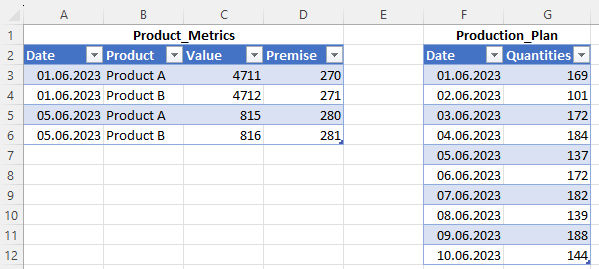Here you can see the two tables in Excel

# My goal

I want to create the following table in Power BI Desktop: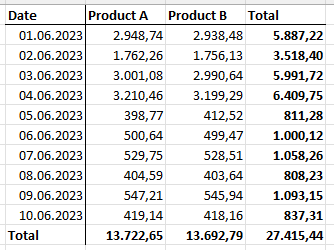I have replicated the result I want to achieve once in Excel

The formula for this is actually quite simple. It is:

(Values / Premise) * QuantityIn Excel it is relatively easy to calculate the results

# My data model in Power BI Desktop

In addition to the two tables mentioned above, I also have a date table dimDate in my data model:In the data model I have 4 tables

I also created a smaller version of the Product_Metrics table: Product_Metrics SMALL. This only contains the date column and each date in it only once. I need this table for one of the later calculations.The table "Production_Metrics SMALL"

# My measures

The first measure is very simple:

`Total quantities = SUM(Production_Plan[Quantities])`

The next metric is a bit more involved as I need to output a metric value from Production_Metrics for each date. This is challenging because the table does not contain a value for every date.

`Total of metrics = VAR vDate =LASTNONBLANK(    'Product_Metrics SMALL',    IF(        'Product_Metrics SMALL'[Date] <= MAX(DimDate[Date]),        "yes",        BLANK()    ))VAR vResult =SUMX(    CALCULATETABLE(        Product_Metrics,        Product_Metrics[Date] = vDate,        ALL(DimDate)    ),    Product_Metrics[Value])RETURNvResult`

To show what the measure does, I put the field Production_Metrics[Value] and [Total of metrics] next to each other:[Total of metrics] returns the most recent value for each date

Using the same principle, I create the measure [Total of premise]:

`Total of metrics = VAR vDate =LASTNONBLANK(    'Product_Metrics SMALL',    IF(        'Product_Metrics SMALL'[Date] <= MAX(DimDate[Date]),        "yes",        BLANK()    ))VAR vResult =SUMX(    CALCULATETABLE(        Product_Metrics,        Product_Metrics[Date] = vDate,        ALL(DimDate)    ),    Product_Metrics[Premise])RETURNvResult`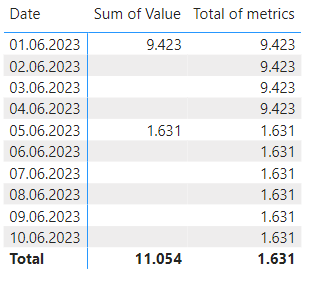[Total of premise] also returns the last valid value.

Now I create the measure in which I do the division and the multiplication:

`Forecast =SUMX(    ADDCOLUMNS(        SUMMARIZE(            DimDate,            DimDate[Date]        ),        "Result",        DIVIDE([Total of metrics], [Total of premise]) * [Total quantities]    ),    [Result])`

Unfortunately, the overall results in the right (red-framed) column are no longer correct:The overall results (outlined in red are incorrect

The wrong results result because Power BI first calculates the sums and then divides them. I present this for June 1st, 2023:

This is what Power BI calculates:

(4.711 + 4.712) / (270 + 271) * 169 = 2.944

Power BI should actually calculate the following:

(4.711 / 270) * 169 = 2.949

(4.712 / 271) * 169 = 2.938

2.949 + 2.938 = 5.877

Of course, the reason Power BI isn't calculating the way I want it to is that there is no filter context for the Production_Metrics[Product] field in the Totals column. I am now trying to solve this problem with the following measure:

`Forecast 2 =SUMX(    ADDCOLUMNS(        SUMMARIZE(            NATURALLEFTOUTERJOIN(                DimDate,                Product_Metrics            ),            DimDate[Date],            Product_Metrics[Product]        ),        "Metrics", [Total of metrics],        "Premisse", [Total of premise],        "Quantities", [Total quantities]    ),    DIVIDE([Metrics], [Premisse]) * [Quantities])`

My idea here is to do a LEFT OUTER JOIN of the dates table with the Product_Metrics table so that all the dates are preserved. At the same time, I use SUMMARIZE to create a filter context for the dimDate[Date] and Product_Metrics[Product] fields.

Unfortunately, this measure now only gives results for the two days on which there are values for Product_Metrics[Value] and Product_Metrics[Premise].Now dates are missing in the matrix

Who can help?

1 ACCEPTED SOLUTIONSuper User

I think you can simplify things a fair bit 🙂

I just mocked up an example using your data (from the tables at the top of your post).

PBIX attached

(Note: This differed slightly from the Excel calcs you showed later - I think there is a typo in Product B Premise on 5 June in the subsequent Excel calculations).

Here's my suggestion:

1. Create data model as follows, with a 'Product' dimension added: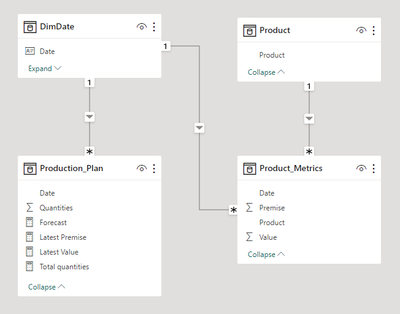2. Create base measures as follows:

``````Latest Premise =
LASTNONBLANKVALUE (
DimDate[Date] <= MAX ( DimDate[Date] ),
SUM ( Product_Metrics[Premise] )
)

Latest Value =
LASTNONBLANKVALUE (
DimDate[Date] <= MAX ( DimDate[Date] ),
SUM ( Product_Metrics[Value] )
)

Total quantities =
SUM ( Production_Plan[Quantities] )``````

3. Create forecast measure that sums (Value/Premise) * Quantity by Product/Date

``````Forecast =
SUMX (
-- Sum over Date & Product
GENERATE ( VALUES ( DimDate[Date] ), VALUES ( 'Product'[Product] ) ),
DIVIDE ( [Latest Value], [Latest Premise] ) * [Total quantities]
)``````

4. Visualise Forecast by Product/Date in matrix: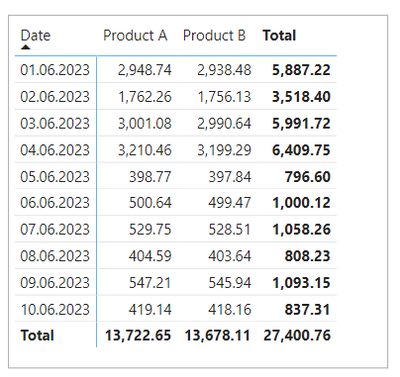Note: You may want to add conditions that blank out the Latest Value and Latest Premise measures for dates later than the latest date in the fact tables. This is not needed for the Forecast measure since the Quantity measure is blank for such dates anyway.

Does this work in your model?

Regards

Owen Auger
Blog
3 REPLIES 3Helper III

Hello @OwenAuger, thank you for your response!

I'll take a look at your file and give you feedback.Super User

I think you can simplify things a fair bit 🙂

I just mocked up an example using your data (from the tables at the top of your post).

PBIX attached

(Note: This differed slightly from the Excel calcs you showed later - I think there is a typo in Product B Premise on 5 June in the subsequent Excel calculations).

Here's my suggestion:

1. Create data model as follows, with a 'Product' dimension added:2. Create base measures as follows:

``````Latest Premise =
LASTNONBLANKVALUE (
DimDate[Date] <= MAX ( DimDate[Date] ),
SUM ( Product_Metrics[Premise] )
)

Latest Value =
LASTNONBLANKVALUE (
DimDate[Date] <= MAX ( DimDate[Date] ),
SUM ( Product_Metrics[Value] )
)

Total quantities =
SUM ( Production_Plan[Quantities] )``````

3. Create forecast measure that sums (Value/Premise) * Quantity by Product/Date

``````Forecast =
SUMX (
-- Sum over Date & Product
GENERATE ( VALUES ( DimDate[Date] ), VALUES ( 'Product'[Product] ) ),
DIVIDE ( [Latest Value], [Latest Premise] ) * [Total quantities]
)``````

4. Visualise Forecast by Product/Date in matrix:Note: You may want to add conditions that blank out the Latest Value and Latest Premise measures for dates later than the latest date in the fact tables. This is not needed for the Forecast measure since the Quantity measure is blank for such dates anyway.

Does this work in your model?

Regards

Owen Auger
BlogHelper III

@OwenAuger, that was exactly what I was looking for! Not only did you provide me with the solution, you also simplified the other calculations considerably!
Thank you very much!
Best regards from GermanyAnnouncements#### Power BI Monthly Update - November 2023

Check out the November 2023 Power BI update to learn about new features.#### Fabric Community News unified experience

Read the latest Fabric Community announcements, including updates on Power BI, Synapse, Data Factory and Data Activator.#### The largest Power BI and Fabric virtual conference

130+ sessions, 130+ speakers, Product managers, MVPs, and experts. All about Power BI and Fabric. Attend online or watch the recordings.Top Solution Authors
Top Kudoed Authors
Users online (2,941)